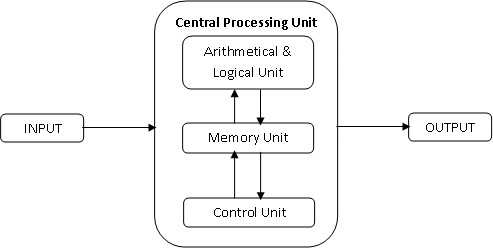## Computer

A computer is an electronic device which takes input, processes the input, and produces the output. Of course, same definition will be applicable for any electronic device. "Compute" means performing a mathematical operation. Since computer performs the computations, it's been called as "Computer".

## Architectural Diagram of a Computer

Computer is broadly divided into several different units• Input Unit
• Central Processing Unit
• Arithmetical and Logical Unit
• Control Unit
• Memory Unit
• Output Unit

### Input Unit

Input Unit will be useful to take input from the user. There are various different input devices that are available in the market now. But the very common input devices are Keyboard and Mouse.

### Central Processing Unit

This is the very important unit of a computer. Whole processing taken place in this unit. This unit can be classified into three different units.

ALU: ALU is an acronym of Arithmetical and Logical Unit. All the mathematical operations (Addition, Subtraction, Multiplication, Division) and logical operations (AND, OR, NOT) are performed here.

MU: MU is the short form for Memory Unit. Computer records the information here i.e.; this is the computer brain.

CU: Control Unit takes care of organizing the coordination between all the other units. i.e.; it takes the input and sends to ALU if any mathematical or logical operations are needed and sends to MU if the information is to be stored and finally sends the information to Output Unit

### Output Unit

Output Unit is to display the output to the user. This is the end result of an operation/problem. There are various different output devices available in the market but the very commonly used output devices are Monitor and Printer.